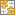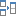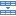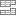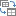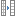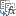# How to calculate days between dates in Excel

This tutorial will teach you a few quick and easy ways to find out how many days are between two dates in Excel.

Are you wondering how many days between two dates? Maybe, you need to know the number of days between today and some date in the past or future? Or, you just want to count working days between two dates? Whatever your problem is, one of the below examples will certainly provide a solution.

## Days between dates calculator

If you are looking for a quick answer, just supply the two dates in the corresponding cells, and our online calculator will show you how many days there are from date to date:

Curious to know the formula that has calculated your dates? It's as simple as `=B3-B2` :)

Below you will find the detailed explanation on how this formula works and learn a few other methods to calculate days between dates in Excel.

## How many days between dates calculation

The easiest way to calculate days between dates in Excel is by subtracting one date from another:

For example, to find out how many days are between dates in cells A2 and B2, you use this formula:

`=B2 - A2`

Where A2 is an earlier date, and B2 is a later date.

The result is an integer that represents no. of days between two dates: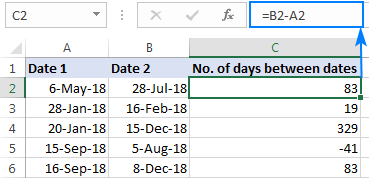### How this formula works

As you probably know, Microsoft Excel stores dates as serial numbers starting on 1-Jan-1900, which is represented by the number 1. In this system, 2-Jan-1900 is stored as the number 2, 3-Jan-1900 as 3, and so on. So, when subtracting one date from another, you actually subtract the integers representing those dates.

In our example, the formula in C3, subtracts 43226 (the numeric value of 6-May-18) from 43309 (the numeric value of 28-Jul-18) and returns a result of 83 days.

The beauty of this method is that it works perfectly in all cases, no matter which date is older and which is newer. If you are subtracting a later date from an earlier date, like in row 5 in the screenshot above, the formula returns a difference as a negative number.

## Calculate number of days between dates in Excel with DATEDIF

Another way to count days between dates in Excel is by using the DATEDIF function, which is specially designed to work out the date difference in various units, including days, months and years.

To get the number of days between 2 dates, you supply the start date in the first argument, end date in the second argument, and "d" unit in the last argument:

DATEDIF(start_date, end_date, "d")

In our example, the formula goes as follows:

`=DATEDIF(A2, B2, "d")`

Unlike the subtraction operation, a DATEDIF formula can only subtract an older date from a newer date, but not the other way round. If the start date is later than the end date, the formula throws a #NUM! error, like in row 5 in the screenshot below: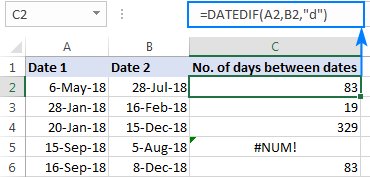Note. DATEDIF is an undocumented function, meaning it is not present in the list of functions in Excel. To build a DATEDIF formula in your worksheet, you will have to type all the arguments manually.

## Count days between dates with Excel DAYS function

The users of Excel 2013 and Excel 2016 have one more amazingly simple way to calculate days between two dates - the DAYS function.

Please pay attention that compared to DATEDIF, a DAYS formula requires the arguments in the reverse order:

DAYS(end_date, start_date)

So, our formula takes the following shape:

`=DAYS(B2, A2)`

Like subtraction, it returns the difference as a positive or negative number, depending on whether the end date is greater or smaller than the start date: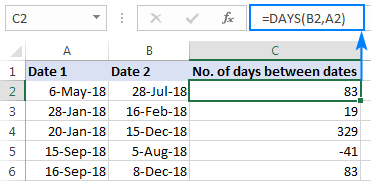## How to calculate number of days between today and another date

In fact, calculating the number of days from or before a certain date is a particular case of "how many days between dates" math. For this, you can use any of the formulas discussed above and supply the TODAY function instead of one of the dates.

To calculate the number of days since date, i.e. between a past date and today:

TODAY() - past_date

To count the number of days until date, i.e. between a future date and today:

Future_date - TODAY()

As an example, let's calculate the difference between today and an earlier date in A4:

`=TODAY() - A4`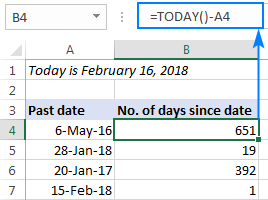And now, let's find out how many days are between today and a later date: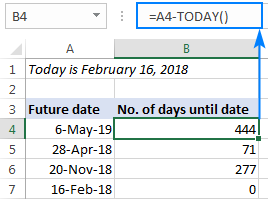## How to calculate working days between two dates in Excel

In situations when you need to get the number of working days between two dates, use the NETWORKDAYS function:

NETWORKDAYS(start_date, end_date, [holidays])

The first two arguments should already look familiar to you, and the third (optional) argument allows excluding a custom list of holidays from the day count.

To find out how many working days are between two dates in columns A and B, use this formula:

`=NETWORKDAYS(A2, B2)`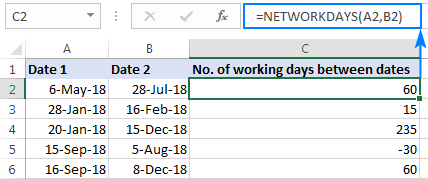Optionally, you can enter your holiday list in some cells and tell the formula to leave out those days:

`=NETWORKDAYS(A2, B2, \$A\$9:\$A\$10)`

As the result, only business days between two dates are counted: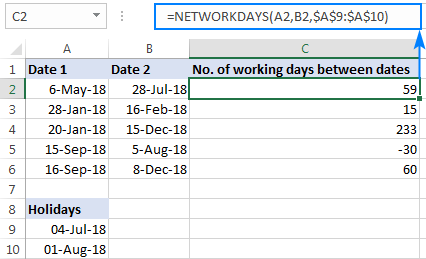Tip. In case you need to handle custom weekends (e.g. weekends are Sunday and Monday or Sunday only), use the NETWORKDAYS.INTL function, which allows you to specify what days of the week should be considered weekends.

## Find number of days between two dates with Date & Time Wizard

As you see, Microsoft Excel provides a handful of different ways to count days between dates. If you are not sure which formula to use, let our Date & Time Wizard do the how-many-days-between-two-dates calculation for you. Here's how:

1. Select the cell in which you want to insert the formula.
2. On the Ablebits Tools tab, in the Date & Time group, click Date & Time Wizard: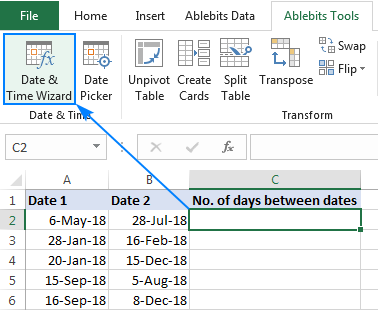3. In the Date & Time Wizard dialog window, switch to the Difference tab and do the following:
• In the Date 1 box, enter the first date (start date) or a reference to the cell containing it.
• In the Date 2 box, enter the second date (end date).
• In the Difference in box, select D.

The wizard immediately shows a formula preview in the cell and the result in the Difference in box.

4. Click the Insert formula button and have the formula inserted in the selected cell. Done!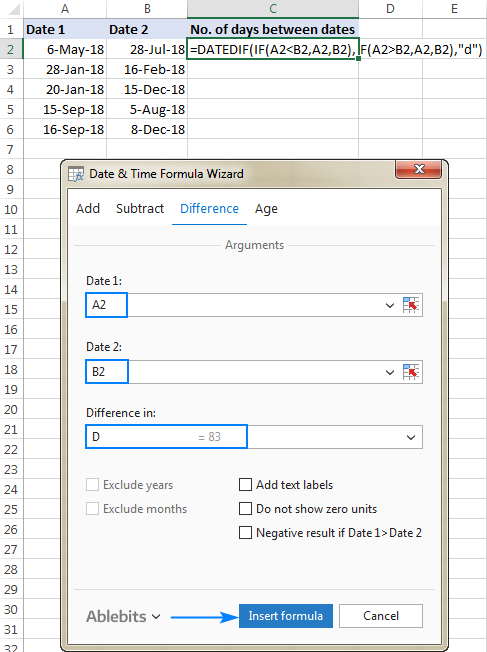A double-click on the fill handle, and the formula gets copied across the column:To display the date difference in a slightly different way, you are free to choose any of the additional options:

• Show text labels - the word "days" will appear along with the number, like shown in the screenshot below.
• Do not show zero units­ - if the date difference is 0 days, an empty string (blank cell) will be returned.
• Negative result if Date 1 > Date 2 - the formula will return a negative number is the start date is later than the end date.

The screenshot below shows a couple of additional options in action: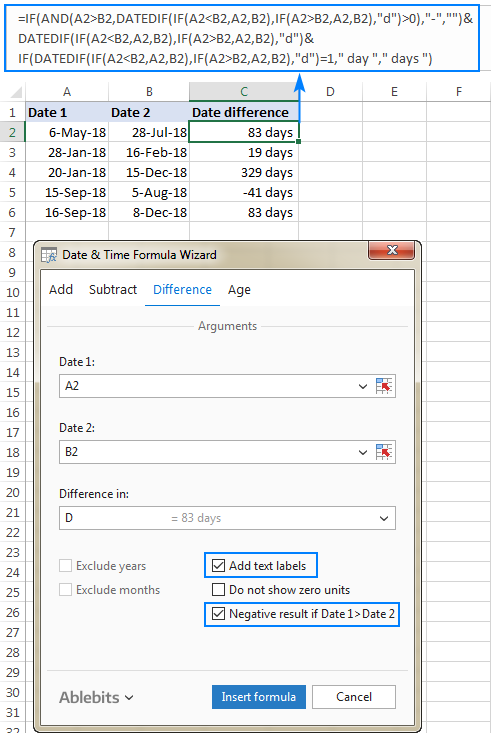This is how you calculate the number of days between dates in Excel. If you'd like to test our Date & Time Formula Wizard in your worksheets, you are welcome to download 14-day trial version of Ultimate Suite, which includes this as well as 60+ other time-saving tools for Excel. If you like the tools and decide to get a license, don't miss a special opportunity that we provide exclusively to our blog readers:

Get Promo Code for Ultimate Suite - exclusive offer for our blog readers!

How Many Days Between Dates - examples (.xlsx file)
Ultimate Suite - trial version (.zip file)

### 65 responses to "How to calculate days between dates in Excel"

1.Nitesh says:

how to calculate times difference.
if start date and start time in different column and end date and end time are in different column than how to calculate.

2.Doug says:

Nitesh:
The formula is ((End Date + End Time)-(Start Date + Start Time))*24
Format each cell the way you usually do for each variable.
Format the result cell as General or Number.

3.Anandhi Subramani says:

Very informative and useful for me.

Thank you.

4.Matt says:

In testing, it appears the DATEDIF does not compute date values prior to Jan 1, 1900. What's the solution for computing date different (age, for example), if the birth date is in the late 1800s?

5.Niju says:

Hi my question is i want value against date 20/09/2018 Subtract 18/09/2018 in excel

6.Date says:

Can you please tell how can I add a text in this for example tell me the formula for current date to my excel data date is greater than 5 days I need one text as abcd.. can you please help me in this formula

7.need a date says:

Could you please help me with the formula to work out how many days between dates, but if there is no start date (yet) to display 0 instead of 43388

•Mary Trifuntova (Ablebits.com Team) says:

Hello,
=IF(ISBLANK(A1), 0, DATEDIF(A1,B1,"d"))

Hope you’ll find this information helpful.

•Pranav Jadhav says:

When one column date is 30/6/2019 and once column is blank (no date) then how to check difference between two columns , i need result "0" if any formula try to check two columns difference

•Steev says:

Excellent, thank you; just what I needed - the date difference and a zeroed-out field while only the start date is currently known.

•MB says:

Thank you Mam..

8.krishanu says:

I would like to subtract Sundays from the networking days calculated

9.prema says:

I need to know the day of the date in one coloumn
for Example 23/10/2018 what would be the Day?

For the 100 lines i will have the dates, i need to find the day

•Mary Trifuntova (Ablebits.com Team) says:

Hello, Prema.
Thank you for contacting us and for your question.

If you need to replace 23/10/2018 with the corresponding day of the week, you just need to select your cells, then press Ctrl+1 to open the Format Cells dialog. On the Number tab, select Custom from the Category list and type the following date format: dddd

Hope you’ll find this information helpful.

10.Danielle says:

Hi

I am trying to total the number of days a certain job takes to do. However if the task is done in the same day how do I get excel to count this as 1 day?

Any feedback would be super - thank you
Danielle

•Mary Trifuntova (Ablebits.com Team) says:

Hi Danielle,

Assuming that your dates and in A and B columns, the formula below should work for you:
=IF(B1 = A1, 1, B1-A1)

Just enter this formula in C1 and then copy it along the column.

If you have any questions, don't hesitate to ask.

•Renee says:

What if I wanted to use this formula with "B1" being "Today"? I can't seem to make that work.

11.victoria catterall says:

Hi,

I'm trying to do something similar to Danielle, but would like to calculate the number of days something is open, so I would need to use 3 dates to calculate this, date open, date closed and today.

Would anyone be able to help with the formula?

Thanks Vicky

12.MOHAMMAD ABID QURAISHI says:

Dear SIr,
Please share demo how to do like this because i trying so many time but i not get actual formula so i request to you share on video to how make formula this is very valuable formula.
Thanks & Best Regards

13.Sid says:

Hi,
I have entered entry and leaving dates from India for last 3 years, and have found the number of days in between. I want this for a range of dates, example in 2017, how many days was I in the country? Pls help, thanks.

14.Asif says:

Dear sir,
I want to calculate days from date to end date i.e if vacation start from 10 jan to 20 jan, by above formula it will show 10 days but atual it is 11 days. Can you explain please

15.Violin_Fish says:

I need a formula to calculate number of days between 2 dates. However, the number of days must be separated. Example: column A: 29/1/19 - 2/2/19, then column B = number of days for January, column C = number of day for February.

16.Mike says:

Hi...is there a way to Calculate the # of workdays between 2 dates & then allocate them by month? I just can't seem to get any formula to work?

17.Tena Franc says:

Hi I am really stryggling with the correct formulas for these columns. I think I have them done correctly, but can't seem to figure out how to get the "Days Complete" and the "Days Remaining" in real time. So if it is completed, it should show 0, and if compared to the present I don't want it to show a negative number but just a zero for completion. HELP PLEASE!! Here are my columns and their values:

START DATE 12/18 DAY OF MONTH* 18 END DATE 1/4 DURATION*(WORK DAYS) 14 DAYS COMPLETE* 14 DAYS REMAINING*-56 TEAM MEMBER ABC PERCENT COMPLETE ?

18.Thshara Bandara says:

Count days from today. If the call is blank, shows nothing.

19.Thshara Bandara says:

Hi, I need help if someone knows about excel formulas. I have an excel sheet that has an ordered date and days been ordered. But some of the cells do not have requested date, in that case, I need the cells to be empty.

20.Biswajit Routray says:

I want formula for counting days between two dates. In case of from date and to date are same so here the number of days is 1. But all above formula shows 0.

•Rahul says:

=IF(AND(A=E,B=F,C=G),1,difference formula)
here A,B,C contains date 1 and E,F,G contains second date.

21.Favour says:

Please i need help,find the number of days between two dates

22.Jay says:

I need help with calculating the number of holidays in a per month.
These are the holidays for 2019:
1-Jan-19
9-Apr-19
18-Apr-19
1-May-19
5-Jun-19
These are the holiday for 2020:
1-Jan-20
9-Apr-20
10-Apr-20
1-May-20
24-May-20

23.Maria Khan says:

I need to prepare a summary of different workers. how i calculate in a single formula that how many workers we have staff cadre and are regular and contract and how many staff are regular and contract.

24.Himanshu Dave says:

Hello,,
Good Morning.
I need Deviation in days between Actual date and Desired date as per Planner,,
Example..
Cell A1 is last calibration date (22 August 2019)
Cell B1 is Next Calibration date (22 Sept 2019) calibration validity is 1 month.
Cell A 10 is calibration done date (27 sept 2019)
how to calculate the calibration deviation in the planner,,

25.dharmesh says:

I need to include start date as well end date so what to do. I just did A2-B2+1. any other formula

26.Ruta says:

This counter is not that good... He counts first and last day. And then it is not correct. For example I need to count 90 days from 17 nov. Last day I get 15 febr. But it is not right - then I have 91 day!!! He should not count the last day...

27.Max says:

If the date format in your excel sheet does not reflect what is displayed on your windows taskbar, then this may not work for you. In order to fix this you can change your Regional format data with the following instructions:
Right-click on time and choose "adjust date/time"
Under related settings choose "Date, time, & regional formatting"
Under Regional format data choose "Change data formats"
Select the short date format that works for you
Bask in the glory of solving this mystery that plagued me for longer than I care to mention.

28.Paige says:

Hi
I need to enter a third date into the argument. I am counting the number of days elapsed between a date in the past (say in cell A1) and 'TODAY' (say the formula is in Cell A2) , but I then need to add in a completion date (say in cell A3) and need the number of days to stop counting once the completion date is entered. How do I tell the formula to stop counting when that completion date is entered? Oh, I also need to tell the formula that if A1 is blank, leave A2 blank. So at the moment my formula would look like this:
=IF(ISBLANK(A1),"",(TODAY()-A1))

29.Elizabeth.Samantha says:

Positive thinking leads to positive life, and negative thinking leads to negative life

30.Marie says:

What's the formula if I want to know the age of an invoice as of today?
A1 - 9-dec-2019 (invoice date)
B1 - 9-Jan-2020 (invoice due date)
c1 - age as of Jan 30 (date today)
Thanks for the help in advance

31.Excel Query says:

i have a query:
i want to calculate the no of days starting from say 1st Jan, 12 AM midnight( start of day) to 5th Jan, 12 AM midnight (end of day).
this should give me 5 days but the excel formula gives me 4 days
how do i calculate that?

32.Excel Query says:

Hi there,
It appears that DATEIF doesn't work in excel online - is there an alternate formula
Cheers

33.entire column says:

i have to do this =b2-a2 formula for over a 1000 lines. how do i do this for the entire column?

34.RAJIV RANJAN KUMAR says:

hi
wow networkdays is properly working.
thank you

35.Ajay says:

Hi There,
My question is if I want to know the days gap between 2 dates, but it should not calculate the first day. From the the second day the counting should begin.

Here is a query -
Start Date 10-March-2020
End Date 15-March-2020
Leaving Date 11-March-2020, counting should begin from 12-March-2020.

36.charmi says:

date + days = count date
02/06/20 + 5 = 06/06/20

•charmi says:

plz help for how to cakculate ??

•Alexander Trifuntov (Ablebits.com Team) says:

Hello!
Your date 02/06/20 in its entirety looks like this: 02/06/20 00:00:00. Therefore, when you add 5 days, one of them is 02/06/2020. And then add another 4 days. We get 06/06/20

37.Felicia says:

Hi, Hope someone could help.
I have 2 days the same date.
The difference of days will be Zero, 0.
What I want is to days to todate.
For instance,
A1 B1 C1
3/29/2012 3/29/2012 A1-B1 + days till today(for instance 26 July 2020)
Hope someone could help.
Thank you,
Felicia

•Alexander Trifuntov (Ablebits.com Team) says:

Hello Felicia!
I don't quite understand what your problem is. Read the section "How many days between dates calculation" above. Please specify what you were trying to find, what formula you used and what problem or error occurred. Give an example of the source data and the expected result.
It’ll help me understand it better and find a solution for you.

38.Kathryn says:

I have 5 customer transactions, each on different days, displayed on different rows, there is a common customer ID - i want to understand how many days from the earliest transaction date to the most recent transaction date. ie. Calculating customer lifetime in days. Help? Thanks!

•Kathryn says:

Note - i could have 20k rows of customer data so cannot manually take dates to calculate. Needs to be something like IF Customer ID = "Customer ID", calculate days between Newer Date and Older Date...

•Alexander Trifuntov (Ablebits.com Team) says:

Hello Kathryn!
Unfortunately, without seeing your data it is impossible to give you advice.

I'm sorry, it is not very clear what result you want to get. Could you please describe your task in more detail and send us a small sample workbook with the source data and expected result to support@ablebits.com? Please shorten your tables to 10-20 rows/columns and include the link to your blog comment.

39.Lisa says:

I have four columns with dates. First column in date of receipt(7/1/20), second column is date of 1st response (7/5/20), third column is IF a 2nd response is needed and fourth column is IF a third response is needed. I need a column that totals # of days from date of receipt until last response (whether 1, 2, or 3). How do I build a formula for that?

•Alexander Trifuntov (Ablebits.com Team) says:

Hello!
If I understand your task correctly, the following formula should work for you:

=MAX(A1:D1)-A1

Hope this is what you need.

40.Saleemchd says:

I want a formulae to count every month as 30 days.

•Alexander Trifuntov (Ablebits.com Team) says:

I’m sorry but your task is not entirely clear to me. For me to be able to help you better, please describe your task in more detail. Please specify what you were trying to find, what formula you used. Give an example of the source data and the expected result.
It’ll help me understand it better and find a solution for you.

41.Innocent Athanasi says:

V4-INT((V4-WEEKDAY(K4)+1)/7)-1

i have come across this formula, i just need to understand how exactly it works.

V4= column containing the Difference between two dates.
K4= column containing different dates and times.

kindly help.

42.Kathy Dunn says:

I would like a formula to calculate 21 working days from any given date, can you help please

43.Pallavi Alloju says:

As an example, let's calculate the difference between today and an earlier date in A4:

=TODAY() - A4

From the above example I am trying to calculate the number of working days.
The date Column1
21/10/2020 In this column I would like to display the number of working days from 24/10/2020 till today

44.Douglas says:

In excel 2007 database.
I have current date in column A, there are also dates in columns AH, AX, BI and BS.
It would be very useful if I can replace date with days between A and each of the other 4. so number of days between A and AH, A and AX, A and BI, and A and BS. Since there are in excess of 30000 rows, it would be useful to automatically calculate the days for each row with a single formula!
Found your articles very informative and hope you can assist with this rather strange request.
Many thanks in anticipation,
Stay safe,
Regards,
Doug

•Alexander Trifuntov (Ablebits.com Team) says:

Hello!
Unfortunately, you can only replace the date in a cell with the number of days using a VBA macro. If a cell contains a date, then it is no longer possible to write a formula to it.

45.SUNIL KUMAR SAHU says:

Sir I want to Calculate number of days between start date & time to end date & time i.e 20-11-2020 07:00 am to 21-11-2020 07:00 am 1 day if date 21-11-2020 07:01 am then 2 days it will show if DATE IS 20-11-2020 time is any time after 07:00 am to 21-11-2020 07:00 am it calculate 1 days after 07:00 am it calculate 2 days automatically can you help me ?

•Alexander Trifuntov (Ablebits.com Team) says:

Hello!
What formula are you using? What format is set in a cell with a formula? Remember that dates and times are recorded in Excel as numbers.

Ultimate Suite for Microsoft Excel